# PPM tests

## October 7, 2015

After the last post, I discovered a couple of bugs in the reconstruction techniques that were leading to very poor internal energy solutions on the square wave test. The results have improved considerably, but there is still some assymmetry, which is especially pronounced when using third order reconstruction (PPMP or PPMC). This post investigates the assymetries in the high mach number square wave test.

First of all, is the assymetry caused by another bug? To test this, I run the same tests, but with the velocity reversed.

Figure 1a: PPMP, exact solver, dual energy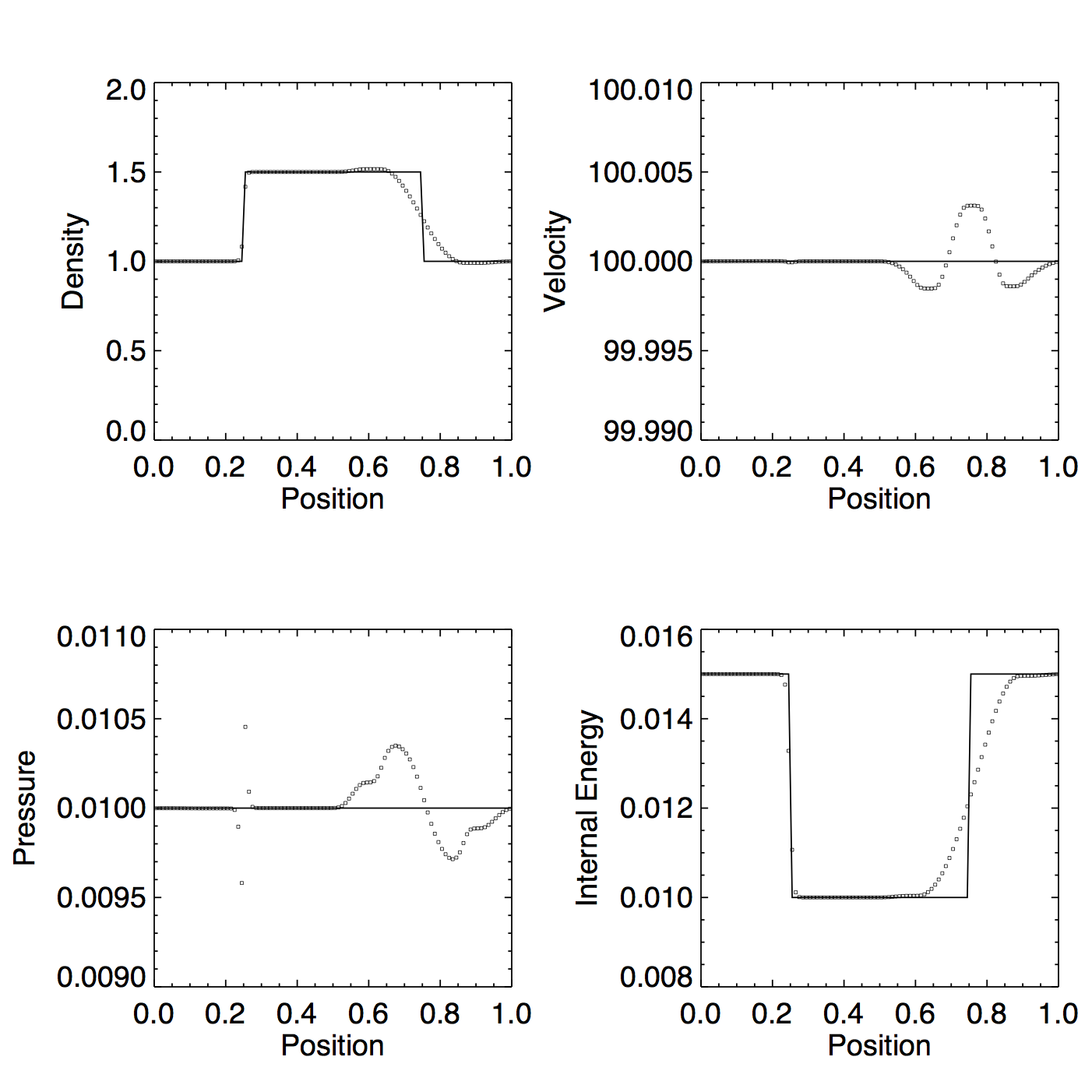Figure 1b: PPMP, exact solver, dual energy, reversed velocity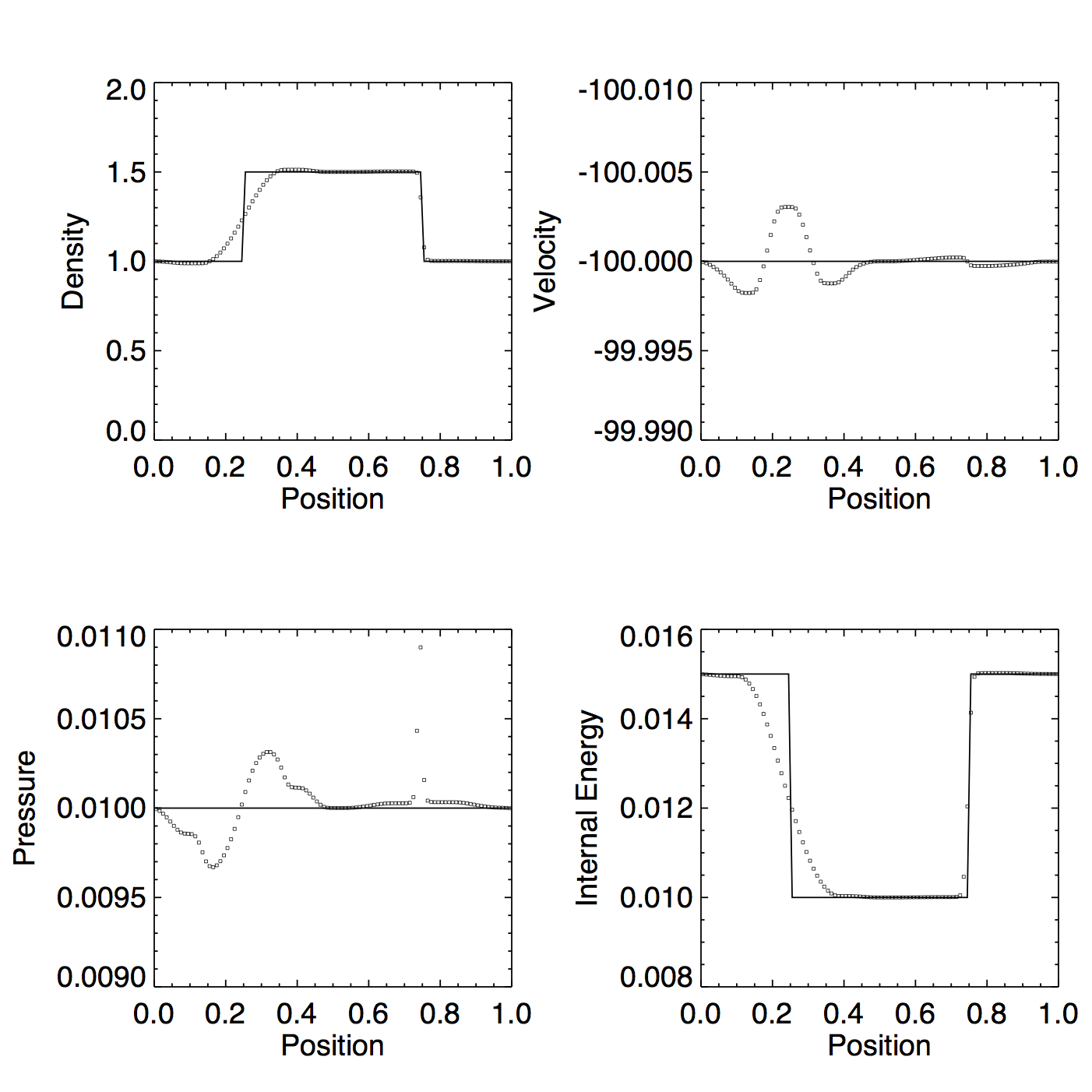Figure 2a: PPMC, exact solver, dual energy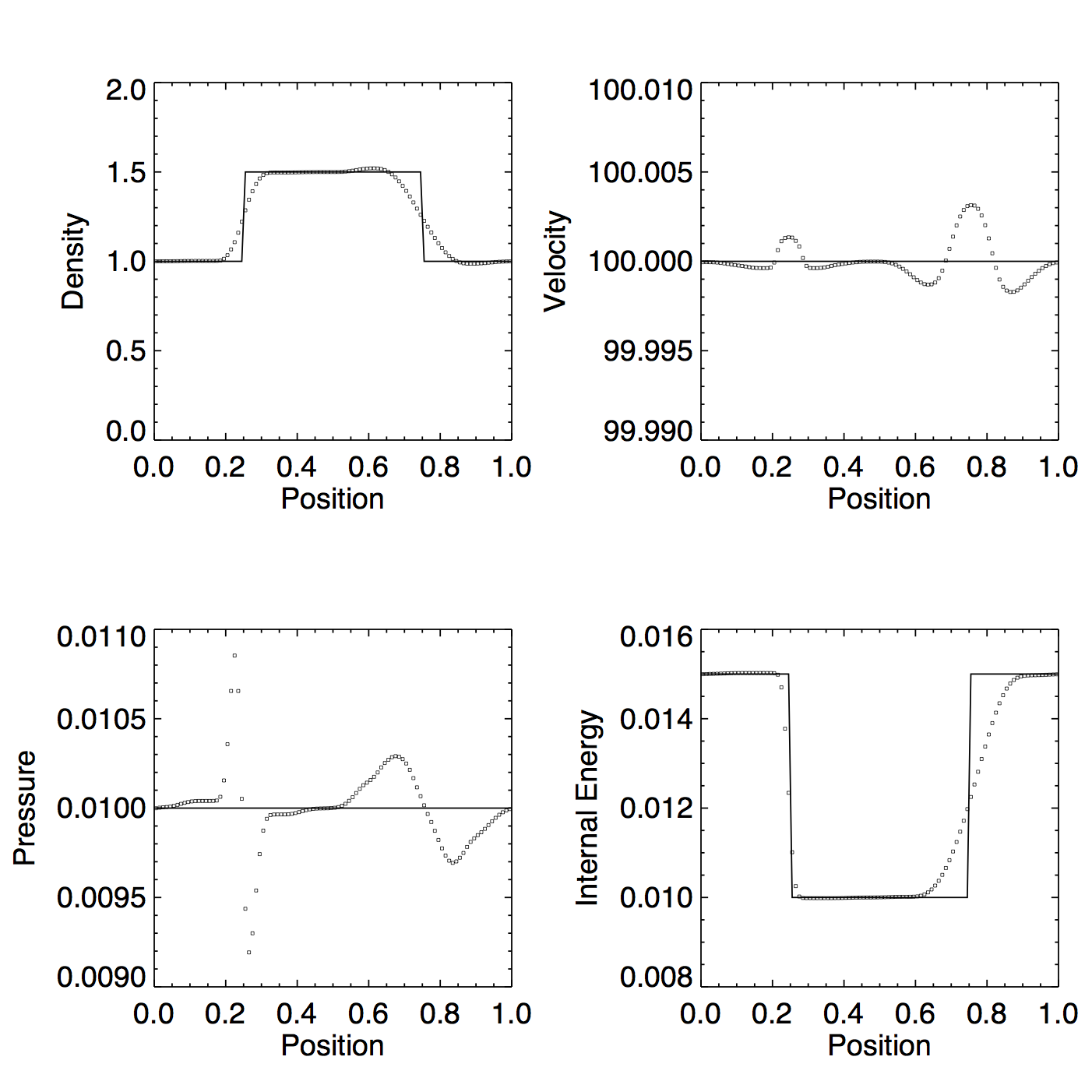Figure 2b: PPMC, exact solver, dual energy, reversed velocity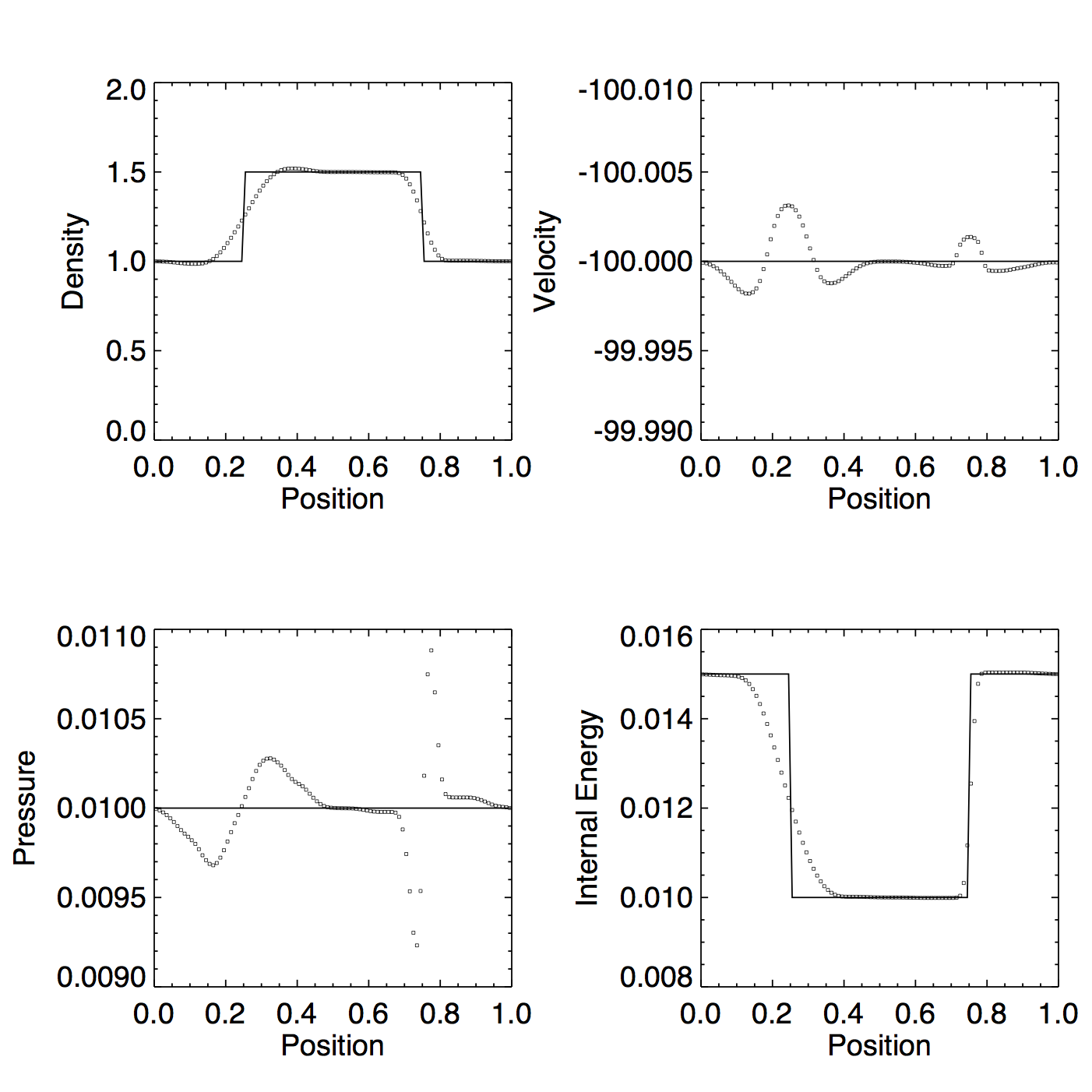The behavior is very similar for the reversed tests, but clearly not identical. In particular, the pressure in the PPMP tests is underestimated in one direction but not the other.

Instead of using the advected internal energy to calculate the flux ($F(e) = \bar{\rho}\bar{v}\bar{e}$, with $\bar{e} = e_{L}$ if $\bar{v} > 0$, $\bar{e} = e_{R}$ if $% $), what happens if I use the sampled pressure ($F(e) = \bar{p}\bar{v} / (\gamma - 1)$)?

Figure 3a: PPMP, exact solver, dual energy, sampled e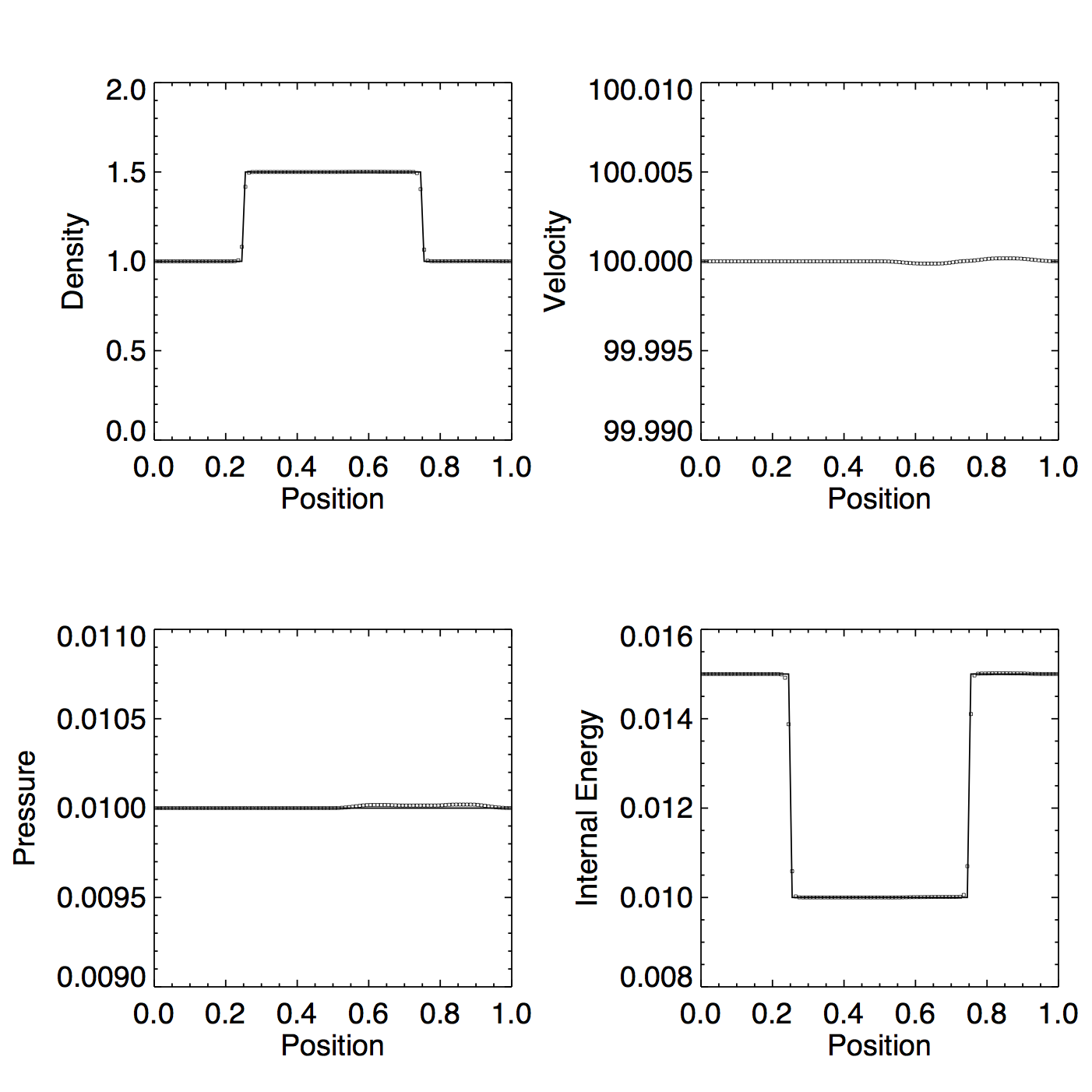Figure 3b: PPMc, exact solver, dual energy, sampled e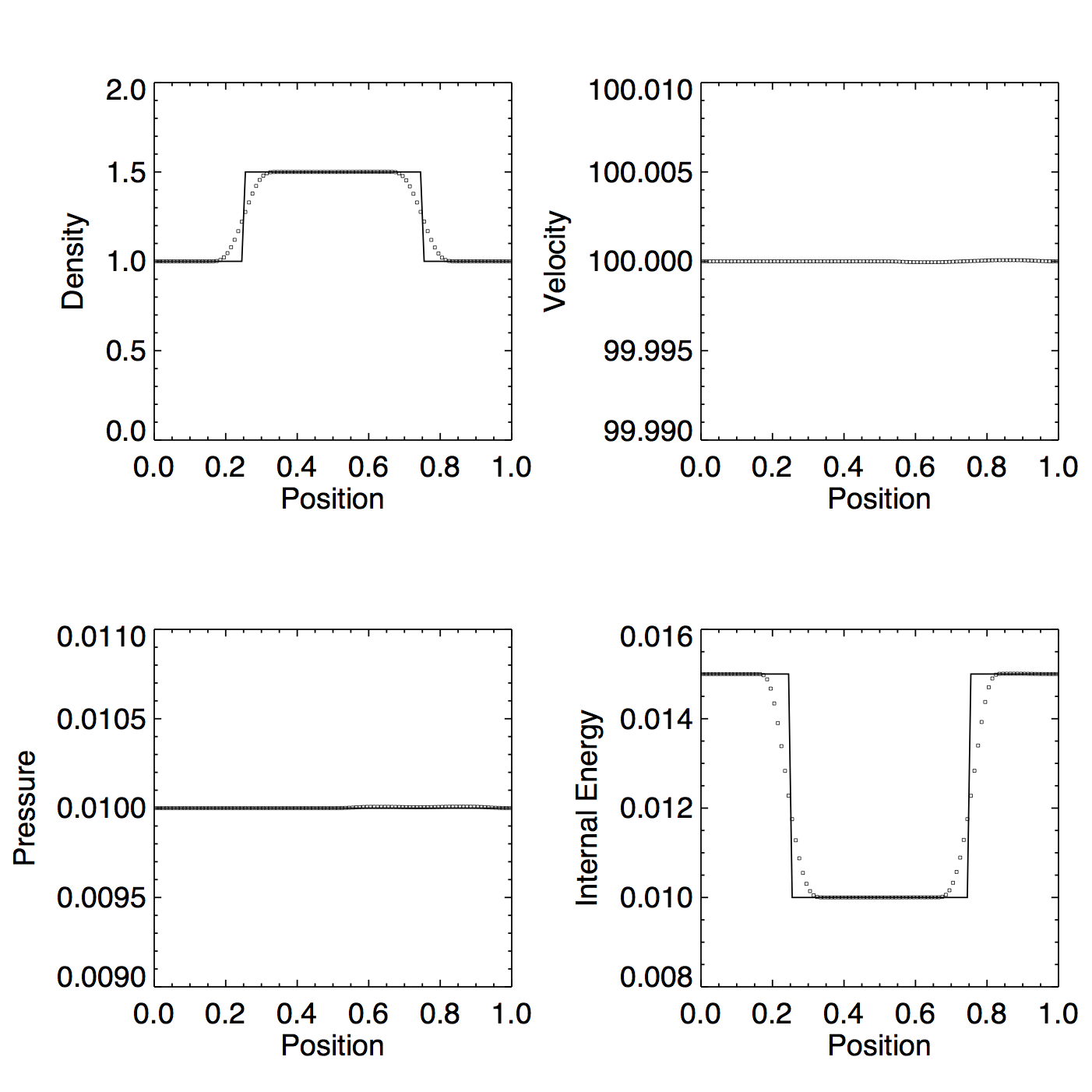This certainly helps, but I’m not sure it would work in a scenario where dual energy is actually needed.